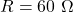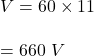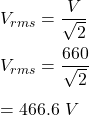## The maximum current output of a 60 omega circuit is 11 A. What is the rms voltage of the circuit?

Question

The maximum current output of a 60 omega circuit is 11 A. What is the rms voltage of the circuit?

in progress 0
6 months 2021-08-24T12:16:21+00:00 1 Answers 2 views 0

## Answers ( )

V = 466.6 volts

Explanation:

Given that

Resistance,Current, T = 11 A

We know that,

Voltage, V = IR

So,The rms voltage of the circuit is :So, the rms voltage of the circuit is equal to 466.6 volts.